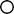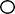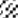## GATE 2021 Statistics Previous Year Paper

GATE 2021 Statistics Previous Year Paper

General Aptitude (GA)

Q.1 – Q.5 Multiple Choice Question (MCQ), carry ONE mark each (for each wrong answer: – 1/3).

Q.6 – Q. 10 Multiple Choice Question (MCQ), carry TWO marks each (for each wrong answer: – 2/3).

##### Q.1 – Q.9 Multiple Choice Question (MCQ), carry ONE mark each (for each wrong answer: – 1/3).

Q.10 – Q.25 Numerical Answer Type (NAT), carry ONE mark each (no negative marks).

Q.26 – Q.43 Multiple Choice Question (MCQ), carry TWO mark each (for each wrong answer: – 2/3).

Q.44 – Q.55 Numerical Answer Type (NAT), carry TWO mark each (no negative marks).

## GATE 2021 Production and Industrial Engineering Previous Year Paper

GATE 2021 Production and Industrial Engineering Previous Year Paper

General Aptitude (GA)

Q.1 – Q.5 Multiple Choice Question (MCQ), carry ONE mark each (for each wrong answer: – 1/3).

Q.6 – Q. 10 Multiple Choice Question (MCQ), carry TWO marks each (for each wrong answer: – 2/3).

Production & Industrial Engineering (PI)

1. – Q.17 Multiple Choice Question (MCQ), carry ONE mark each (for each wrong answer: – 1/3).Q.18 – Q.25 Numerical Answer Type (NAT), carry ONE mark each (no negative marks).

Q.26 – Q.36 Multiple Choice Question (MCQ), carry TWO mark each (for each wrong answer: – 2/3).

Q.37 – Q.55 Numerical Answer Type (NAT), carry TWO mark each (no negative marks).

*MTA means Marks to All

## GATE 2021 Physics Previous Year Paper

##### General  Aptitude (GA)

Q.1 – Q.5 Multiple Choice Question (MCQ), carry ONE mark each (for each wrong answer: – 1/3).

Q.6 – Q. 10 Multiple Choice Question (MCQ), carry TWO marks each (for each wrong answer: – 2/3)

##### Physics (PH)
1. – Q.9 Multiple Choice Question (MCQ), carry ONE mark each (for each wrong answer: – 1/3).

Q.10 – Q.16 Multiple Select Question (MSQ), carry ONE mark each (no negative marks).

Q.17 – Q.25 Numerical Answer Type (NAT), carry ONE mark each (no negative marks).

Q.26 – Q.41 Multiple Choice Question (MCQ), carry TWO mark each (for each wrong answer: – 2/3).

Q.42 – Q.46 Multiple Select Question (MSQ), carry TWO mark each (no negative marks).

## GATE 2021 Petroleum Engineering Previous Year Paper

GATE 2021 Petroleum Engineering Previous Year Paper

General Aptitude (GA)

Q.1 – Q.5 Multiple Choice Question (MCQ), carry ONE mark each (for each wrong answer: – 1/3).

1. 6 – Q. 10 Multiple Choice Question (MCQ), carry TWO marks each (for each wrong answer: – 2/3).##### Petroleum Engineering (PE)

Q.1 – Q.13 Multiple Choice Question (MCQ), carry ONE mark each (for each wrong answer: – 1/3).

Q.14 – Q.19 Multiple Select Question (MSQ), carry ONE mark each (no negative marks

Q.26 – Q.37 Multiple Choice Question (MCQ), carry TWO mark each (for each wrong answer: – 2/3)

Q.38 – Q.39 Multiple Select Question (MSQ), carry TWO mark each (no negative marks).

Q.40 – Q.55 Numerical Answer Type (NAT), carry TWO mark each (no negative marks).

*MTA means Marks to All

## GATE 2021 Metallurgical Engineering Previous Year Paper

##### General Aptitude (GA)

Q.1 – Q.5 Multiple Choice Question (MCQ), carry ONE mark each (for each wrong answer: – 1/3).

Q.6 – Q. 10 Multiple Choice Question (MCQ), carry TWO marks each (for each wrong answer: – 2/3).

##### Metallurgical Engineering (MT)

Q.1– Q.13 Multiple Choice Question (MCQ), carry ONE mark each (for each wrong answer: – 1/3).

Q.14 – Q.25 Numerical Answer Type (NAT), carry ONE mark each (no negative marks).

Q.26 – Q.36 Multiple Choice Question (MCQ), carry TWO mark each (for each wrong answer: – 2/3

Q.37 – Q.55 Numerical Answer Type (NAT), carry TWO mark each (no negative marks).

## GATE 2021 Mining Engineering Previous Year Paper

##### Q.1 – Q.5 Multiple Choice Question (MCQ), carry ONE mark each (for each wrong answer: – 1/3).

Q. 6 – Q. 10 Multiple Choice Question (MCQ), carry TWO marks each (for each wrong answer: – 2/3).

##### Mining Engineering (MN
1. – Q.19 Multiple Choice Question (MCQ), carry ONE mark each (for each wrong answer: – 1/3).

Q.26 – Q.36 Multiple Choice Question (MCQ), carry TWO mark each (for each wrong answer: – 2/3).